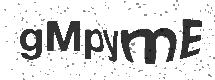Multiplication and division

• Recall multiplication and division facts for multiplication tables up to 12 × 12
• Use place value, known and derived facts to multiply and divide mentally, including: multiplying by 0 and 1; dividing by 1; multiplying together 3 numbers
• Recognise and use factor pairs and commutativity in mental calculations
• Multiply two-digit and three-digit numbers by a one-digit number using formal written layout
• Solve problems involving multiplying and adding, including using the distributive law to multiply two-digit numbers by 1 digit, integer scaling problems and harder correspondence problems such as n objects are connected to m objects

### Get in touch### Connect with us

Ludlow Junior School

Peveril Rd,
Woolston,
Southampton,
Hampshire,
SO19 2DW

T: 023 8044 7885

E: This email address is being protected from spambots. You need JavaScript enabled to view it.